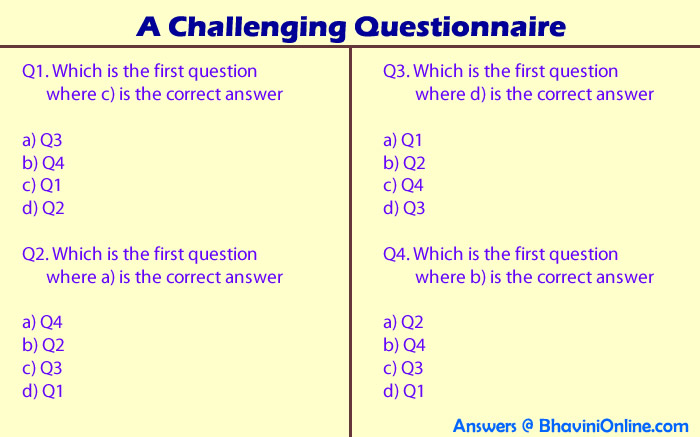# A Challenging Questionnaire

Here’s a challenging questionnaire build to confuse you. So don’t get lost with the questions and make sure you answer them correctly.

The actual order of the multiple choice questions are as follows;

a) Q1

b) Q2

c) Q3

d) Q4

Q1. Which is the first question where c) is the correct answer

a) Q3

b) Q4

c) Q1

d) Q2

Q2. Which is the first question where a) is the correct answer

a) Q4

b) Q2

c) Q3

d) Q1

Q3. Which is the first question where d) is the correct answer

a) Q1

b) Q2

c) Q4

d) Q3

Q4. Which is the first question where b) is the correct answer

a) Q2

b) Q4

c) Q3

d) Q1